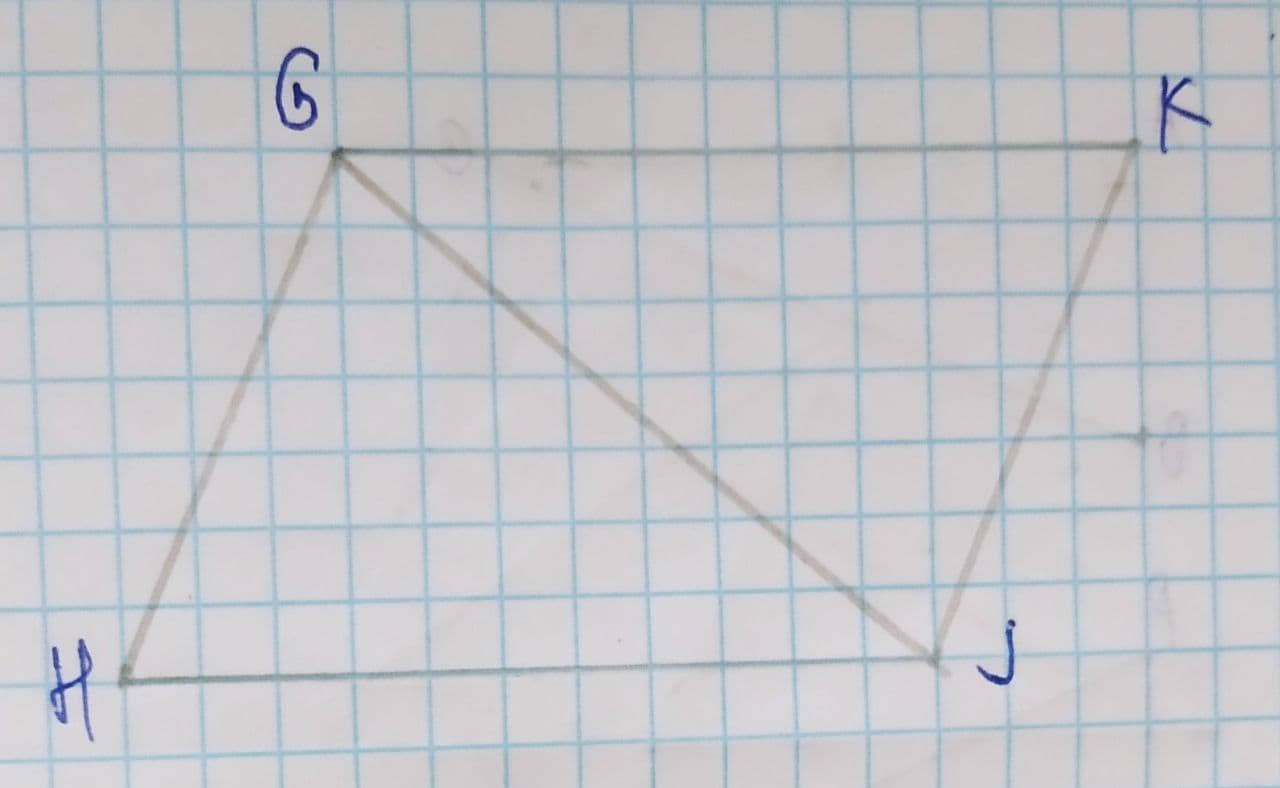Haven

2021-07-26

To show that two sides are congruent.
Given information:
$\mathrm{\angle }HGJ\stackrel{\sim }{=}\mathrm{\angle }KJG$
$\mathrm{\angle }KGJ\stackrel{\sim }{=}\mathrm{\angle }HJG$Luvottoq

Formula used:
The below property is used:
Two triangles are congruent by ASA congruence rule.
Proof:
From figure, we getIt is given that,
$\mathrm{\angle }HGJ\stackrel{\sim }{=}\mathrm{\angle }KJG$
$\mathrm{\angle }KGJ\stackrel{\sim }{=}\mathrm{\angle }HJG$
By reflexive property, we get
$\stackrel{―}{GJ}\stackrel{\sim }{=}\stackrel{―}{GJ}$
By ASA congruence rule, we get
$\mathrm{△}GHJ\stackrel{\sim }{=}\mathrm{△}JKG$
As corresponding parts of congruent triangles are congruent, we get
$\stackrel{―}{HG}\stackrel{\sim }{=}\stackrel{―}{KJ}$

Do you have a similar question?